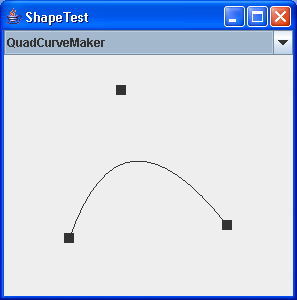# Compares two lines are returns true if they are equal or both null. : Shape « 2D Graphics GUI « Java

Compares two lines are returns true if they are equal or both null.

```

import java.awt.geom.Line2D;

public class Main {

/**
* Compares two lines are returns <code>true</code> if they are equal or
* both <code>null</code>.
*
* @param l1  the first line (<code>null</code> permitted).
* @param l2  the second line (<code>null</code> permitted).
*
* @return A boolean.
*/
public static boolean equal(final Line2D l1, final Line2D l2) {
if (l1 == null) {
return (l2 == null);
}
if (l2 == null) {
return false;
}
if (!l1.getP1().equals(l2.getP1())) {
return false;
}
if (!l1.getP2().equals(l2.getP2())) {
return false;
}
return true;
}

}

```

### Related examples in the same category

 1 Creating Basic Shapes 2 fillRect (int, int, int, int) method draws a solid rectangle 3 Creating a Shape Using Lines and Curves 4 Combining Shapes 5 Draw rectangles, use the drawRect() method. To fill rectangles, use the fillRect() method 6 Draw line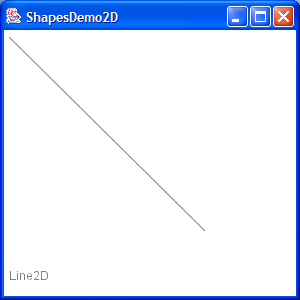7 Draw a Polygon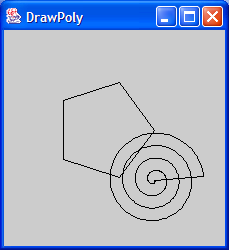8 Draw an oval outline 9 Draw a (Round)rectangle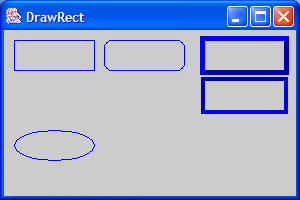10 Fill a polygon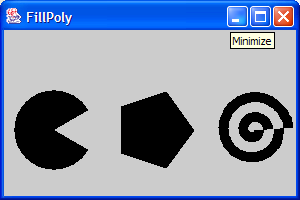11 Fill a solid oval 12 Fill a (Round)rectangle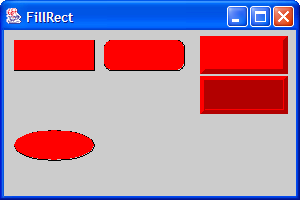13 Change font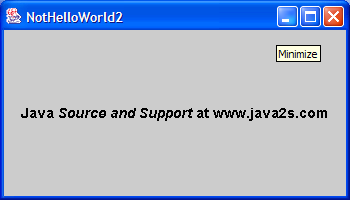14 Draw rectangle 2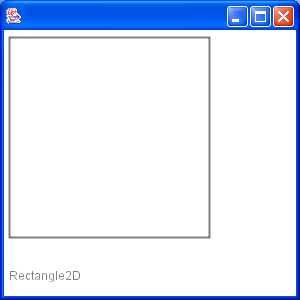15 Draw Arc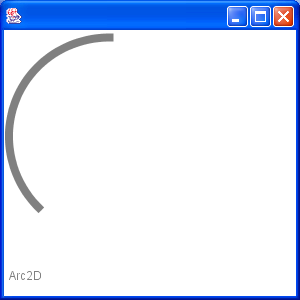16 Draw Ellipse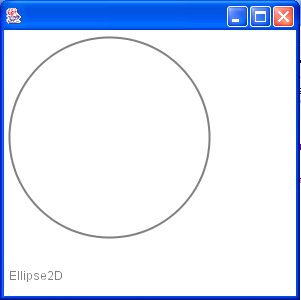17 Fill a Rectangle 2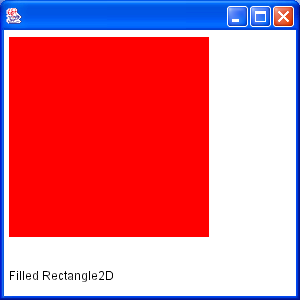18 Fill Arc 2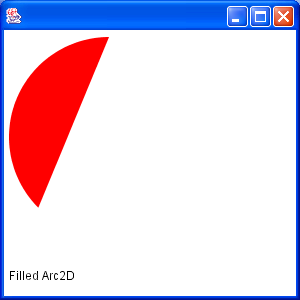19 Draw text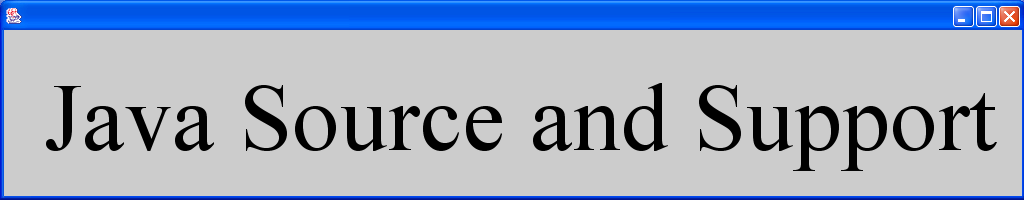20 Draw unicode string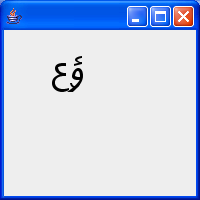21 Shape combine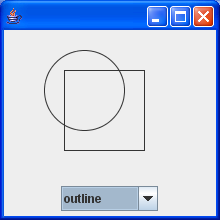22 Effects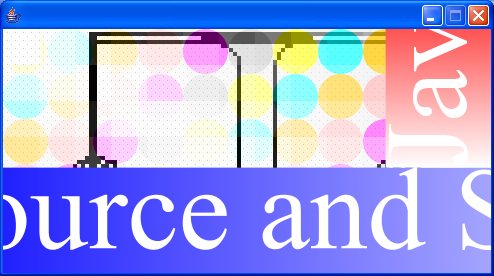23 Mouse drag and drop to draw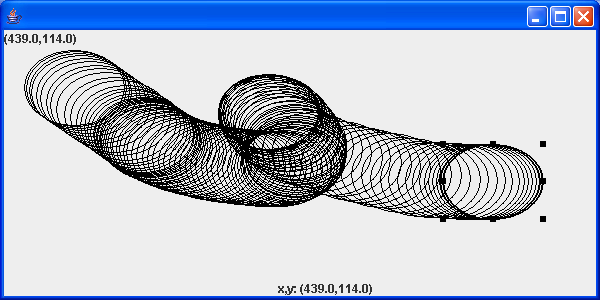24 Arc demonstration: scale, move, rotate, sheer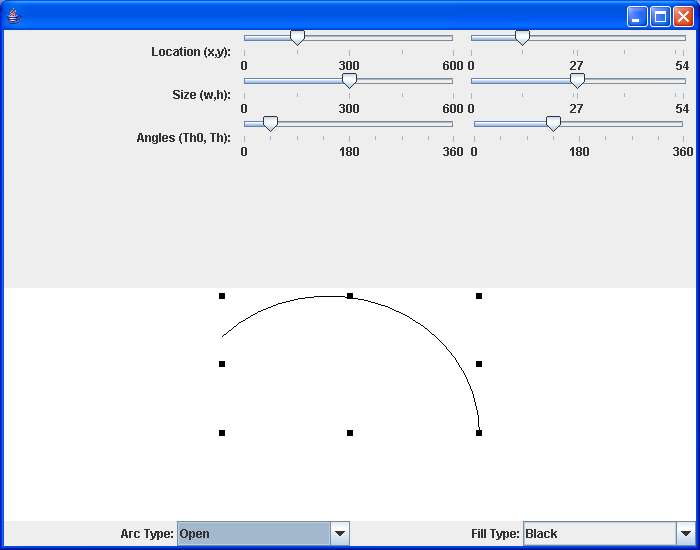25 Hypnosis Spiral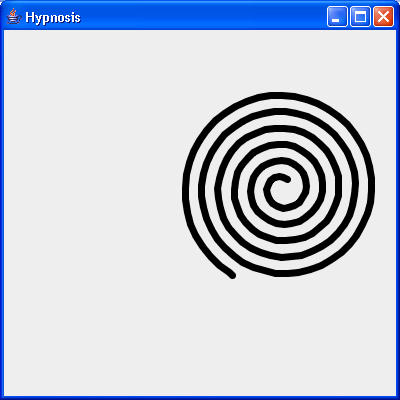26 GlyphVector.getNumGlyphs() 27 Resize a shape 28 Rectangle with rounded corners drawn using Java 2D Graphics API 29 Compares two ellipses and returns true if they are equal or both null. 30 Creates a diagonal cross shape. 31 Creates a diamond shape. 32 Creates a new Stroke-Object for the given type and with. 33 Creates a region surrounding a line segment by 'widening' the line segment. 34 Creates a triangle shape that points downwards. 35 Creates a triangle shape that points upwards. 36 Generate Polygon 37 Polygon with float coordinates. 38 Polyline 2D 39 Serialises a Shape object. 40 Tests two polygons for equality. If both are null this method returns true. 41 Union two rectangles 42 Calculate Intersection Clip 43 Draws a shape with the specified rotation about (x, y). 44 Checks, whether the given rectangle1 fully contains rectangle 2 (even if rectangle 2 has a height or width of zero!). 45 Reads a Point2D object that has been serialised by the writePoint2D(Point2D, ObjectOutputStream)} method. 46 Returns a point based on (x, y) but constrained to be within the bounds of a given rectangle. 47 RectListManager is a class to manage a list of rectangular regions. 48 Fill Rectangle2D.Double and Ellipse2D.Double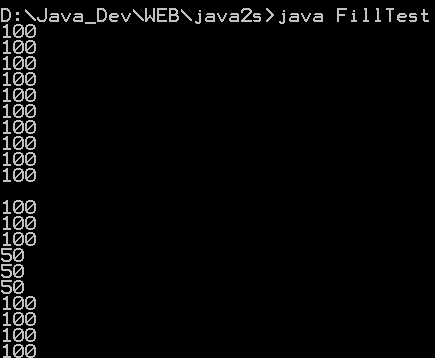49 This program demonstrates the various 2D shapes